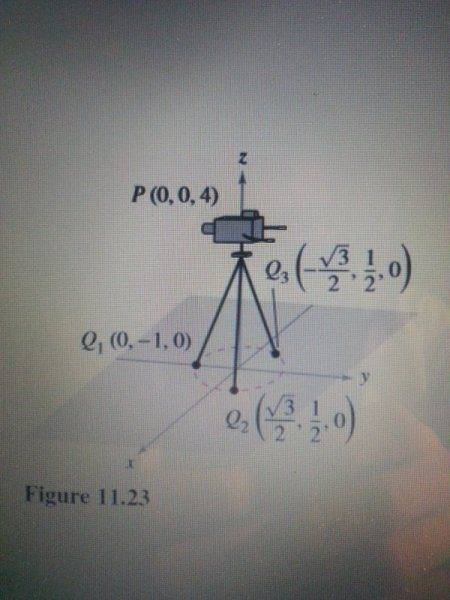# Measuring Vectors## Homework Statement

This is an example problem in my calculus textbook. I don't get how they relate the position vector to the force vector. I have taken a calculus based physic course and I don't remember establishing such relationship between the position and the force vectors.

Note: I have attached a picture of the situation.
[/B]
A television camera weighing 120 pounds is supported by a tripod, as shown in Figure 11.23. Represent the force exerted on each leg of the tripod as a vector.

## Homework Equations

u=cv
v + u= v1 + u1, v2 + u2, v3 + u3
cv=cv1, cv2, cv3

3. The Attempt at a Solution
Let the vectors and represent the forces exerted on the three legs. From Figure 11.23, you can determine the directions of and to be as follows:[/B]

PQ1= <0,-1,-4>
PQ2= <√3/2, 1/2,-4>
PQ3= <-√3/2, 1/2,-4>

Because each leg has the same length, and the total force is distributed equally among the three legs, you know that the magnitude of F1=F2=F3. So, there exists a constant such that

F1= c<0,-1,-4>=F2= c<√3/2, 1/2,-4>=F3= c<-√3/2, 1/2,-4>
Let the total force exerted by the object be given by F= <0,0,-120> Then, using the fact that

F= F1 + F2 + F3

you can conclude that F1, F2, and F3 all have a vertical component of -40. This implies that c(-4)= -40 and c is equal to 10.
Therefore, the forces exerted on the legs can be represented by

F1= <0,- 10, -40>
F2= <5√3, 5, -40>
F3= <-5√3, 5, -40>

Last edited by a moderator:

Orodruin
Staff Emeritus
Homework Helper
Gold Member
2021 Award
The assumption is that the legs only take up forces in the longitudinal direction and therefore must take a force in the direction they are pointing.

The assumption is that the legs only take up forces in the longitudinal direction and therefore must take a force in the direction they are pointing.

I'm sorry, but I am still not very clear on this.

Orodruin
Staff Emeritus